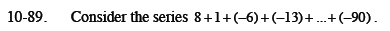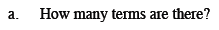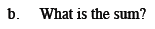Home > CCA2 > Chapter 10 > Lesson 10.2.1 > Problem10-89

10-89.
1. Consider the series 8 + 1 + (−6) + (−13) + … + (−90). Homework Help ✎

1. How many terms are there?

2. What is the sum?Write an expression for the sequence, and find n when t(n) = −90.

15$\text{Use the summation formula }S_n=\frac{n}{2}\left(t\left(1\right)+t\left(n\right)\right)$